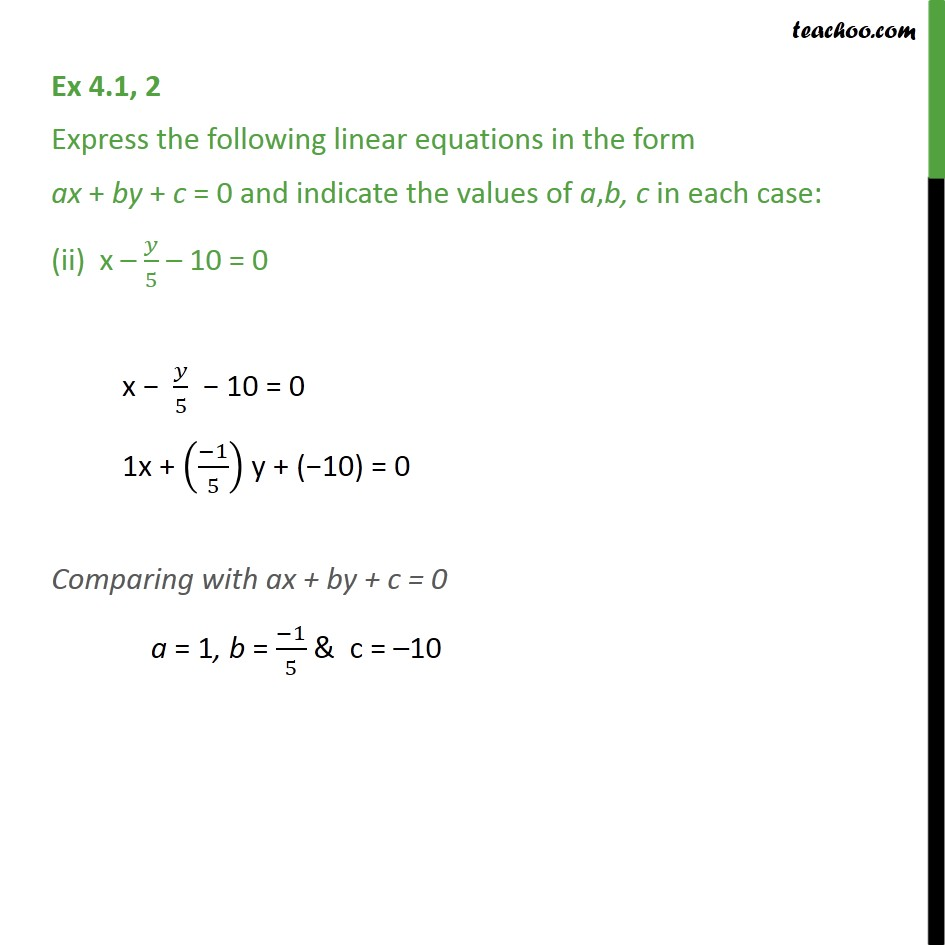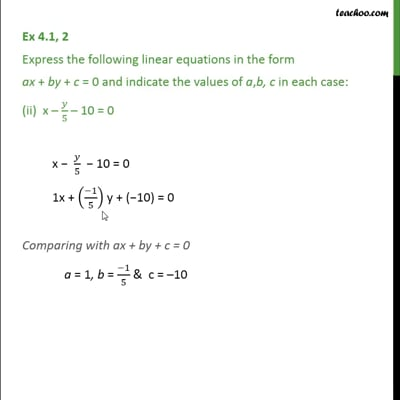Ex 4.1

Chapter 4 Class 9 Linear Equations in Two Variables
Serial order wiseThis video is only available for Teachoo black users

Introducing your new favourite teacher - Teachoo Black, at only ₹83 per month

### Transcript

Ex 4.1, 2 Express the following linear equations in the form ax + by + c = 0 and indicate the values of a,b, c in each case: (ii) x – 𝑦/5 – 10 = 0 x − 𝑦/5 − 10 = 0 1x + ((−1)/5) y + (−10) = 0 Comparing with ax + by + c = 0 a = 1, b = (−1)/5 & c = –10